# Polyhedra

A polyhedron is a figure formed by polygons which enclose a region of $3$ -dimensional space.

The polygons are called faces , the line segments in which they intersect are called edges , and the endpoints of the edges are called vertices .

For example, the pyramid shown below has $7$ faces ( $1$ hexagon and $6$ triangles), $12$ edges (six segments converging at the apex, the point where the side faces meet), and $7$ vertices ( $6$ in the base plus the apex).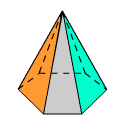A regular polyhedron has for its faces all congruent, regular polygons. There are only five regular polyhedrons; they are known as the platonic solids .

 Name Drawing # of Faces # of Edges # of Vertices Regular Tetrahedron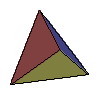$4$ $6$ $4$ Cube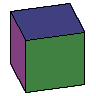$6$ $12$ $8$ Regular Octahedron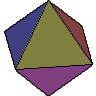$8$ $12$ $6$ Regular Dodecahedron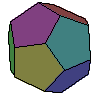$12$ $30$ $20$ Regular Icosahedron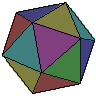$20$ $30$ $12$

You may notice that the number of faces plus the number of vertices, minus the number of edges, is always equal to $2$ . This is true for any polyhedron in $3$ -dimensional space, and is known as Euler's formula :

$V-E+F=2$